# Bihar Board Class 10th Maths Solutions Chapter 13 Surface Areas and Volumes Ex 13.4

Bihar Board Class 10th Maths Solutions Chapter 13 Surface Areas and Volumes Ex 13.4 Textbook Questions and Answers.

## BSEB Bihar Board Class 10th Maths Solutions Chapter 13 Surface Areas and Volumes Ex 13.4Question 1.
A drinking glass is in the shape of a frustum of a cone of height 14 cm. The diameters of its two circular ends are 4 cm and 2 cm. Find the capacity of the glass. S61. Capacity of the glass.
Solution:
V = $$\frac{\pi \times h}{3}$$ x (R² + r² + Rr)
Here, R = 2 cm, r = 1 cm and h = 14 cm. 22
∴ V = $$\frac{\frac{22}{7} \times 14}{3}$$ x (2² + 1² + 2 x 1)cm³
= $$\frac { 44 }{ 3 }$$ x (4 + 1 + 2) cm³
= ($$\frac { 44 }{ 3 }$$ x 7 )cm³ = $$\frac { 308 }{ 3 }$$ cm³ = 102$$\frac { 2 }{ 3 }$$ cm³

Question 2.
The slant height of a frustum of a cone is 4 cm and the perimeters (circumferences) of its circular ends are 18 cm and 6 cm. Find the curved surface area of the frustum.
Solution:
Slant height l = 4 cm (Given)2πr1 = 6 gives πr1 = 3
and, 2πr2 = 18 gives πr2 = 9
Curved surface area of the frustum
= (πr1 + πr2)l
= (3 + 9) x 4 cm²
= 12 x 4 cm²
= 48 cm².Question 3.
A fez, the cap used by the Turks, is shaped like the frustum of a cone (see figure). If its radius oh the open side is 10 cm, radius at the upper base is 4 cm and its slant height is 15 cm, find the area of material used for making it.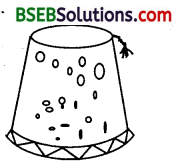Solution:
Here, R = 10 cm, r = 4 cm and l = 15 cm.
Area of the material used for making the fez = Curved surface area of frustum + The surface area of top circular section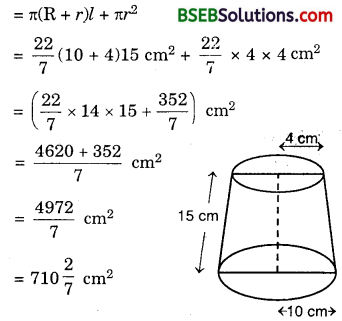Question 4.
A container opened from the top and made up of a metal sheet, is in the form of a frustum of a cone of height 16 cm with radii of its lower and upper ends as 8 cm and 20 cm, respectively. Find the cost of milk which can completely fill the container, at the rate of Rs 20 per litre. Also, find the cost of metal sheet used to make the container, if it costs Rs 8 per 100 cm². (Take π = 3.14)
Solution:
Here, R – 20 cm, r = 8 cm and h = 16 cm.
Capacity of the container = Volume of the frustum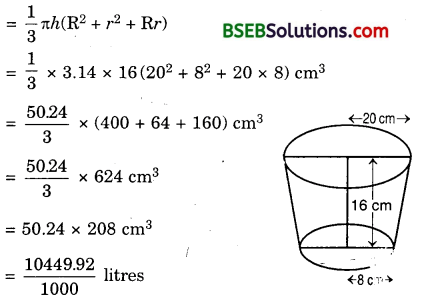Cost of milk at the rate of Rs 20 per litre
= Rs (20 x $$\frac { 10449.92 }{ 1000 }$$ = Rs 208.99 ≈ Rs 209
To find the slant height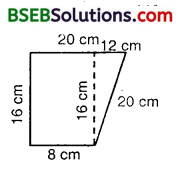l = $$\sqrt{16^{2}+12^{2}}$$ cm
= $$\sqrt{256+144}$$ cm
= $$\sqrt{400}$$ cm = 20 cm
Curved surface area
= π (R + r)l
= $$\frac { 22 }{ 7 }$$ x (20 + 8) x 20 cm² = $$\frac { 22 }{ 7 }$$ x 28 x 20 cm²
= 1758.4 cm²
and area of the bottom
= πr² = $$\frac { 22 }{ 7 }$$ x (8)² cm² = $$\frac { 22 }{ 7 }$$ x 64 cm² = 200.96 cm²
∴ Total area of metal required
= 1758.4 cm² + 200.96 cm²
= 1959.36 cm²
Cost of metal sheet used to manufacture the container at the rate of Rs 8 per 100 cm²
= Rs $$\frac { 8 }{ 1000 }$$ x 1959.36) = Rs 156.75Question 5.
A metallic right circular cone 20 cm high and whose vertical angle is 60° is cut into two parts at the middle of its height by a plane parallel to its base. If the frustum so obtained be drawn into a wire of diameter $$\frac { 1 }{ 16 }$$ cm, find the length of the wire.
Solution:
Let ABC be the metallic cone and DECB be the equired frustum.
Let the two radii of the frustum be DO’ = r2 and BO = r1
From the ∆s ADO’ and ABO,
r2 = h1 tan 30°
= 10 x $$\frac{1}{\sqrt{3}}$$r1 = (h1 + h2) tan 30°
= 20 x $$\frac{1}{\sqrt{3}}$$
Volume of the frustum DBCE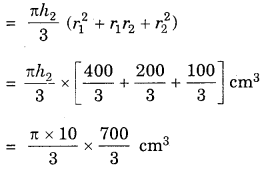Volume of the wire of length l and diameter d
= π($$\frac { d }{ 2 }$$)² x l = $$\frac{\pi d^{2}}{4}$$ x l [V = πr²h]
∴ Volume of the frustum = Volume of the wire drawn from it